Next: Chaotic and rigid dendrites Up: Dendrites Previous: Monotone equivalence and monotone

## Omiljanowski dendrite

The Omiljanowski dendrite has been constructed by K. Omiljanowski in [Charatonik 1991, Example 6.9, p. 182] (see also [Charatonik 1991, Remark 6.11 and Theorem 6.12, p. 183]). It is defined as the closure of the union of an increasing sequence of dendrites in the plane. We start with the unit straight line segment denoted by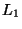. Divideinto three equal subsegments and in the middle of them,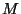, locate a thrice diminished copy of the Cantor ternary set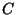. At the midpoint of each contiguous interval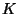to(i.e., a componentof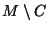) we erect perpendicularly toa straight line segment whose length equals length of. Denote by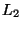the union ofand of all erected segments (there are countably many of them, and their lengths tend to zero). We perform the same construction on each of the added segments: divide such a segment into three equal parts, locate in the middle parta copy of the Cantor setproperly diminished, at the midpoint of any componentofconstruct a perpendicular tosegment as long asis, and denote by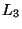the union ofand of all attached segments. Continuing in this manner we get a sequence of dendrites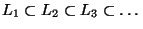. Finally we put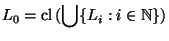and call it a dendrite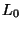. See Figure A.The Omiljanowski dendritehas the following properties, [Charatonik 1991, Example 6.9, p. 182].

1. All ramification points ofare of order 3.
2. The set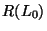of all ramification points ofis discrete (thus nowhere dense).
3. The set of all end points ofis nowhere dense.
4. For each maximal arc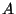inthe closure of the set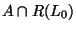contains a homeomorphic copy of the Cantor set.
5.is monotonely equivalent to the dendrite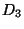.
6. The following conditions are equivalent for a dendrite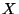(see [Charatonik 1991, Theorem 6.14, p. 185] and [Charatonik et al. 1994, Theorem 5.35, p. 17]; compare [Charatonik et al. 1998, Theorem 2.20, p. 238]):
1.is monotonely equivalent to;
2.is monotonely equivalent to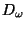;
3.is monotonely equivalent to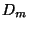for each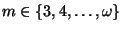;
4.is monotonely equivalent to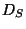for each nonempty set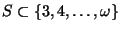;
5.is monotonely equivalent to every dendrite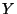having dense set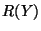of its ramification points;
6.is monotonely equivalent to some dendritehaving dense setof its ramification points;
7.contains a homeomorphic copy of every dendrite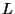such that its set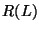of ramification points is discrete and consists of points of order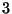exclusively;
8.contains a homeomorphic copy of the dendrite.

According to Property 7 in 1.3.8 (see also Property 6 in 1.3.7) any dendriteis monotonely homogeneous. Another, less restrictive, sufficient condition for monotone homogeneity of a dendrite is the following (see [Charatonik et al. 1997a, Proposition 15, p. 364]).

7. If for a dendritethe set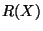of its ramification points is dense in, thenis monotonely homogeneous.

The condition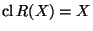, being sufficient, is far from being necessary. Namely the Omiljanowski dendritehas the setdiscrete, and it is monotonely homogeneous. Moreover, the following statement holds, [Charatonik et al. 1997a, Proposition 20, p. 366].

8. If a dendritecontains a homeomorphic copy of, thenis monotonely homogeneous.

It would be interesting to know if the converse to the above statement holds true, i.e., if containing the dendritecharacterizes monotonely homogeneous dendrites. In other words, we have the following question.

Question. Does every monotonely homogeneous dendrite contain a homeomorphic copy of?

The above question is closely related to a more general problem.

Problem. Give any structural characterization of monotonely homogeneous.

Here you can find source files of this example.

Here you can check the table of properties of individual continua.

Here you can read Notes or write to Notes ies of individual continua.Next: Chaotic and rigid dendrites Up: Dendrites Previous: Monotone equivalence and monotone
Janusz J. Charatonik, Pawel Krupski and Pavel Pyrih
2001-11-30